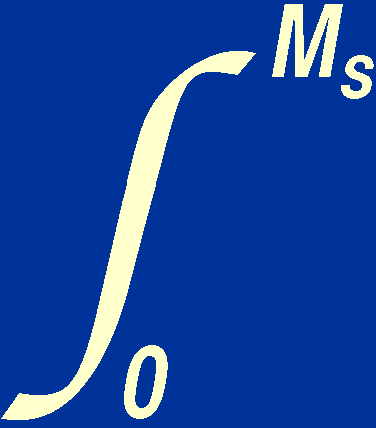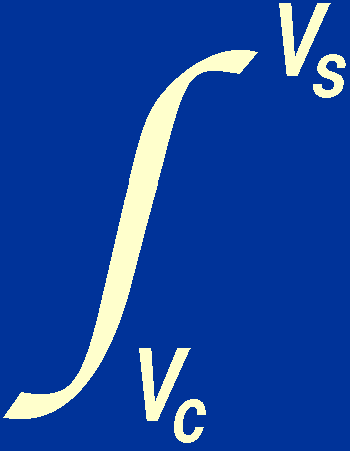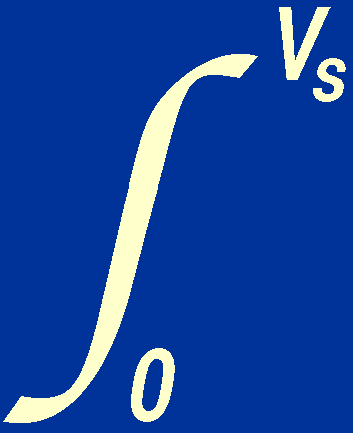One of our immediate aims is to determine the physical state of stellar material, as a knowledge of this is essential if we are to understand stellar structure and evolution. Knowing the minimum central pressure of a star is not enough. We also need to know the density and temperature of stellar material. It is a relatively simple matter to determine the density as we know typical stellar masses and radii. We can determine the temperature by first formulating an important equation known as the virial theorem and then using the virial theorem to calculate the mean temperature of a star.

The virial theorem can be derived as follows. If we divide the equation of hydrostatic support by the equation of mass conservation, we obtain:

(dP / dr) / (dM / dr) = (- GM/ r 2) / 4r 2.

Multiplying both sides of the equation by 4r 3 and rearranging gives:

4r 3 dP = - (GM / r ) dM.

If we now integrate this equation over the whole star, we can write:

3V dP = -(GM / r) dM,

where V is the volume contained within radius r. The left-hand side of the above equation can be integrated by parts, giving:

3 [PV]cs - 3P dV = -(GM / r) dM.

The volume of the star must increase outwards from zero at the centre of the star, i.e. Vc = 0, and we assume that there is a sharp boundary between the surface of the star and the vacuum of space, i.e. Ps = 0. Hence we may write:

3P dV =(GM / r) dM.

Now suppose that a mass M has been assembled into a star of radius r, then the work done ( = force × distance = mass × acceleration × distance) in bringing an additional element of mass dM from infinity to r is:(GM / r 2) dM dr = - (GM / r) dM = d,

where dis the gravitational potential energy of the mass element. The gravitational potential energy of the star is then the integral over M of the above equation,= -(GM / r) dM.

Note that the minus sign here is a matter of convention, where the zero of gravitational potential energy is chosen to be at infinite distance. We could instead have chosen to integrate from r to infinity, in which case the minus sign would disappear. The key point is that the above form of the equation gives the work done, i.e. the energy required, to form the star from its constituent parts. Since assembling a star from its constituent parts at infinity does not require any work to be done, but instead releases energy, the above equation is negative. Had we swapped the integration limits and hence removed the minus sign, the equation would tell us the energy required to disperse a star to infinity, which would clearly require positive work to be done against gravity.

Hence we can write:

3P dV += 0.

This equation, which is of great importance in astrophysics, is known as the virial theorem and, as we shall see when discussing energy generation, it relates the gravitational energy of a star to its thermal energy.